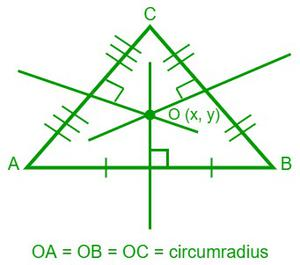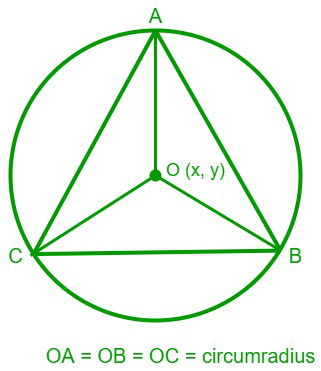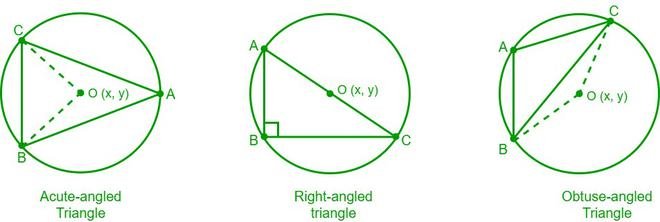# Circumcenter of a Triangle

• Last Updated : 26 May, 2022

The point of intersection, or the meeting point of the perpendicular bisectors of a triangle, is the circumcenter of a triangle and is usually represented by the letter “O”. In simple words, the circumcenter of a triangle is the point of concurrency of the bisectors of the sides of a triangle. The line segment that bisects another line segment at a right angle is called a perpendicular bisector.### What is a Circumcenter?

A circumcenter is the center of a circumcircle, whereas a circumcircle is a circle that passes through all the vertices of a polygon, i.e., a circle in which a polygon is enclosed. Every circle is cyclic, which means every triangle can circumscribe a circle. Hence, any type of triangle will have a circumcenter.

For the construction of the circumcenter of any triangle, the perpendicular bisectors of any two sides of a triangle need to be drawn.

### Properties of a circumcenterLet us consider a triangle ABC. Now, the properties of the circumcenter of a triangle are:

1. The circumcenter of a triangle is equidistant from all the vertices, i.e., OA = OB = OC.
2. All the new triangles that are formed by joining the circumcenter of a triangle to its vertices are isosceles, i.e., ∆ AOB, ∆ BOC, and ∆ COA are isosceles triangles.
3. In a triangle, if ∠A is acute or when O and A are on the same side of BC, then ∠BOC = 2 ∠A.
4. In a triangle, if ∠A is obtuse or when O and A are on different sides of BC, then ∠BOC = 2(180° – ∠A).
5. For an acute-angled triangle, the circumcenter lies inside the triangle.
6. In the case of an obtuse-angled triangle, the circumcenter lies outside the triangle.
7. For a right-angled triangle, the circumcenter lies on the hypotenuse of the triangle.### Construction of the circumcenter of a triangle

To construct the circumcenter of any triangle, we need to draw the perpendicular bisectors of any two sides of the triangle. The following are the steps to construct the circumcenter of a triangle:

Step 1: For the given triangle, draw the perpendicular bisectors of any two sides using a compass.

Step 2: With the help of a ruler, extend the perpendicular bisectors till they intersect at a point.

Step 3: Now, mark the point of intersection, which will be the circumcenter of the given triangle.

We can get the circumcenter accurately by drawing the perpendicular bisector of the third side of the triangle.

### How to find the Circumcenter of a Triangle?

We can calculate the circumcenter of a triangle using different methods.

Method 1

Let us consider a triangle ABC whose vertices are A (x1, y1), B(x2, y2), and C (x3, y3), and O(x, y) is its circumcenter.

Step 1: Calculate the coordinates of the mid-points of the sides AB, BC, and AC using the mid-point formula.

M(xm ,ym) = [(x1 + x2)/2, (y1 + y2)/2]

Step 2: Calculate the slopes of the sides AB, BC, and AC. Let the slope of a side be m, then the slope of its perpendicular bisector is “-1/m”.

Step 3: Now, using the coordinates of the midpoint (xm, ym) and the slope of the perpendicular bisector (-1/m), find out the equation of the perpendicular bisector using the point-slope form.

(y – ym) = -1/m(x – xm)

Step-4: Similarly, find out the equations of the other bisector lines too.

Step-5: Solve any of the two equations and find their intersection point.

The obtained intersection point is the circumcenter of the given triangle.

Method 2

Let us consider a triangle ABC whose vertices are A (x1, y1), B(x2, y2), and C (x3, y3), and O (x, y) is its circumcenter.

We know that the circumcenter of a triangle is equidistant from all the vertices, i.e., OA = OB = OC = circumradius.

Let OA = D1, OB = D2, and OC = D3.

Step-1: Using the distance formula between two coordinates, find the values of D1, i.e.,

(D1)2 = (x – x1)2 + (y – y1)2

Similarly,

(D2)2 = (x – x2)2 + (y – y2)2

(D3)2 = (x – x3)2 + (y – y3)2

Step-2: Now, by equating D1 = D2 = D3 we will get linear equations. By solving these linear equations, we can get the coordinates of the circumcenter O (x, y).

Method-3

Let us consider a triangle ABC whose vertices are A (x1, y1), B (x2, y2), and C (x3, y3), and ∠A, ∠B, and ∠C are their respective angles. O (x, y) is the circumcenter of the triangle.

We can determine the circumcenter easily by using the formula below.

(### Sample Problems

Problem 1: Determine the circumcenter of a triangle with vertices A (1,3), B (0,4), and C (-2,5).

Solution:

Given data,

The vertices of a triangle are A (x1, y1) = (1,3), B (x2 , y2) = (0,4), and C (x3, y3) = (-2,5).

Let “O” be the circumcenter of the triangle ABC and (x, y) be its coordinates.

Let D1 be the distance from the circumcenter to vertex A, i.e., OA = D1.

Let D2 be the distance from the circumcenter to vertex B, i.e., OB = D2.

Let D3 be the distance from the circumcenter to vertex C, i.e., OC = D3.

By using the formula for the distance between two points we get

(D1)2 = (x – x1)2 + (y – y1)2 = (x – 1)2 + ( y – 3)2

(D2)2 = (x – x2)2 + (y – y2)2 = ( x- 0)2 + (y – 4)2

(D3)2 = (x – x3)2 + (y – y3)2 = (x + 2)2 + (y – 5)2

We know that the distances from all the vertices to the circumcenter (O) are equal, i.e.,

OA = OB = OC = circumradius

⇒ D1 = D2 = D3

Now, take D1 = D2

(x -1)2 + (y – 3)2 =  (x – 0)2 + (y -4)2

⇒ x2 – 2x + 1 + y2 – 6y + 9 = x2 + y2 – 8y + 16

⇒ 2x – 2y = -6    ……(1)

Now, take D2 = D3

( x- 0)2 + (y – 4)2 = (x + 2)2 + (y – 5)2

⇒ x2 + y2 – 8y + 16 = x2 + 4x + 4 + y2 – 10y + 25

⇒ 4x – 2y = -13  …..(2)

Now, solve both equations (1) and (2)

2x – 2y = – 6

4x – 2y = -13

(-) (+)      (+)

– 2x = 7

⇒ x = -7/2 = – 3.5

Now, substitute the value of x in equation (1)

2 (-3.5) – 2y = -6

⇒ -7 – 2y = -6

⇒ 2y = -1 ⇒ y = -1/2 = -0.5

Hence, the circumcenter of the triangle ABC is (-3.5, -0.5)

Problem 2:  Determine the circumcenter of the triangle with vertices A (3, -6), B (1, 4), and C (5, 2).

Solution:

Given data,

The vertices of a triangle are A (x1, y1) = (3, -6), B (x2 , y2) = (1, 4), and C (x3, y3) = (5, 2).

Let “O” be the circumcenter of the triangle ABC and (x, y) be its coordinates.

For finding the circumcenter of a triangle, we can calculate the intersection point of any two perpendicular bisectors.

Now, the mid point of the side AB = [(3 + 1)/2, (-6 + 4)/2] = (2, -1)

Slope of AB = (y2 – y1)/(x2 – x1) = (4 + 6)/(1 – 3) = -5

We know that the product of the slopes of two perpendicular lines = -1.

So, the slope of the perpendicular bisector of the side AB = 1/5

Now, the equation of the perpendicular bisector of AB with slope = 1/5 and the coordinates (2,-1) is

(y – (-1)) = (1/5) (x – 2) [From point-slope form]

⇒ 5(y +1) = x – 2

⇒ x – 5y = 7    ……(1)

The mid point of the side BC is [(1 + 5)/2, (4 + 2)/2] = (3,3)

Slope of BC = (y3 – y2)/(x3 – x2) = (2 – 4)/(5 – 1) = -1/2

Now, the slope of the perpendicular bisector of the side BC = 2

The equation of the perpendicular bisector of BC with slope = 2 and the coordinates (3,3) is

(y – 3) = 2(x – 3)  [From point-slope form]

⇒ y – 3 = 2x – 6

⇒ 2x – y = 3    …….(2)

Now, multiply equation (1) with “2” on both sides and subtract equation (2) from the obtained equation.

2x – 10y = 14

2x – y = 3

(-) (+)  (-)

-9y = 11

⇒ y = -11/9

Now substitute the value of y in equation (2)

2x + 11/9 = 3

⇒ x = 8/9

Hence, the circumcenter of the triangle ABC is (8/9, -11/9).

Problem 3: Find the circumcenter of the ∆ ABC with vertices A (1, 3), B (3, 7), and C (5, 9), and the measures of the respective angles are 45°, 45°, and 90°.

Solution:

Given data,

The vertices of a triangle are A (x1, y1) = (0, 3), B (x2, y2) = (3, 7), and C (x3, y3) = (5, 9).

The measures of the angles are ∠A = 45°, ∠B = 45°, and ∠C = 90°.

We know the formula for the circumcenter (O) of a triangle when its vertices and their respective angles are given, i.e.,

Circumcenter (O) =  (,)

O = ()

= ()

= ()

Circumcenter (O) = (1, 3)

Hence, the circumcenter of the triangle ABC is (1,3)

Problem 4: Find the circumcenter of a triangle whose vertices are A (0, 6), B (-8, 4), and C (2, -4).

Solution:

Given data,

The vertices of a triangle are A (x1, y1) = (0, 6), B (x2 , y2) = (-8, 4), and C (x3, y3) = (2, -4).

Let “O” be the circumcenter of the triangle ABC and (x, y) be its coordinates.

For finding the circumcenter of a triangle, we can calculate the intersection point of any two perpendicular bisectors.

Now, the mid point of the side AB = [(0 – 8)/2, (6 + 4)/2] = (-4, 5)

Slope of AB = (y2 – y1)/(x2 – x1) = (4 – 6)/(-8 – 0) = 1/4

We know that the product of the slopes of two perpendicular lines = -1.

So, the slope of the perpendicular bisector of the side AB = -4

Now, the equation of the perpendicular bisector of AB with slope = -4 and the coordinates (-4, 5) is

(y – 5) = -4(x+ 4)  [From point-slope form]

⇒ y – 5 = -4x – 16

⇒ 4x + y = -11     ——— (1)

The mid point of the side BC is [(-8 +2)/2, (4 – 4)/2] = (-3, 0)

Slope of BC = (y3 – y2)/(x3 – x2) = (-4 – 4)/(2 + 8) = -4/5

Now, the slope of the perpendicular bisector of the side BC = 5/4

The equation of the perpendicular bisector of BC with slope = 5/4 and the coordinates (-3, 0) is

(y – 0) = 5/4(x + 3)  [From point-slope form]

⇒ 4y = 5x + 15

⇒ 5x – 4y = -15    ——— (2)

Now, multiply the equation (1) with “4” on both sides and add the result to equation (2).

16x + 4y = -44

5x – 4y = -15

——————

21x = -59 ⇒  x = -59/21

Now, substitute x = -59/21 in equation (1)

4(-59/21) + y = -11

⇒ y = -11 + (236/21) ⇒  y = 5/21

Hence, the circumcenter of the triangle ABC is (-59/21, 5/21)

Problem 5: Find the circumcenter of a triangle whose coordinates are A (3, 8), B (6, 2), and C (-4, 7).

Solution:

Given data,

The vertices of a triangle are A (x1, y1) = (3, 8), B (x2 , y2) = (6, 2), and C (x3, y3) = (-4, 7).

Let “O” be the circumcenter of the triangle ABC and (x, y) be its coordinates.

Let D1 be the distance from the circumcenter to vertex A, i.e., OA = D1.

Let D2 be the distance from the circumcenter to vertex B, i.e., OB = D2.

Let D3 be the distance from the circumcenter to vertex C, i.e., OC = D3.

By using the formula for the distance between two points we get

(D1)2 = (x – x1)2 + (y – y1)2 = (x – 3)2 + ( y – 8)2

(D2)2 = (x – x2)2 + (y – y2)2 = ( x- 6)2 + (y – 2)2

(D3)2 = (x – x3)2 + (y – y3)2 = (x + 4)2 + (y – 7)2

We know that the distances from all the vertices to the circumcenter (O) are equal, i.e.,

OA = OB = OC = circumradius

⇒ D1 = D2 = D3

Now, take D1 = D2

(x -3)2 + (y – 8)2 =  (x – 6)2 + (y -2)2

⇒ x2 – 6x + 9  + y – 16y + 64 = x2 – 12x + 36 + y2 – 4x + 4

⇒ 6x – 4y = -33    …….(1)

Now, take D2 = D3

( x- 6)2 + (y – 2)2 = (x + 4)2 + (y – 7)2

⇒ x2 – 12x + 36 + y2 – 4x + 4 = x2 + 8x + 16 + y2 – 14y + 49

⇒ 20x – 10y = -25

⇒ 4x – 2y = -5    …..(2)

Now, multiply equation (2) with “2” on both sides and subtract the result from the equation (1)

6x – 4y = -33

8x – 4y = -10

(-)  (+)    (+)

-2x = -23 ⇒ x = 23/2 = 11.5

Now, substitute the value of x = 23/2 in equation (2)

4(11.5) – 2y = -5

⇒ 46 – 2y = -5

⇒ 2y = 51

⇒ y = 51/2 = 25.5

Hence, the circumcenter of the triangle ABC is (11.5, 23.5)

My Personal Notes arrow_drop_up